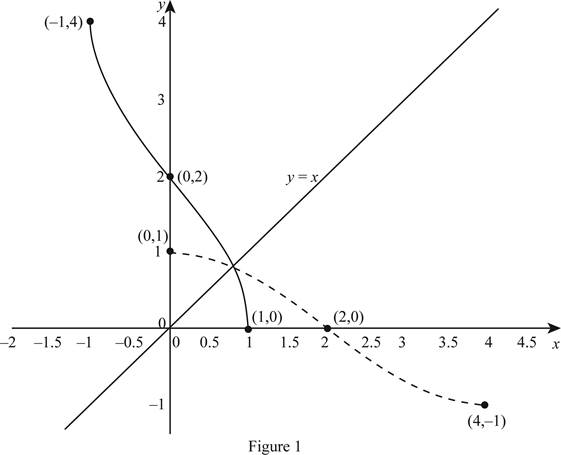# The graph of f − 1 from the given graph f .### Single Variable Calculus: Concepts...

4th Edition
James Stewart
Publisher: Cengage Learning
ISBN: 9781337687805### Single Variable Calculus: Concepts...

4th Edition
James Stewart
Publisher: Cengage Learning
ISBN: 9781337687805

#### Solutions

Chapter 1.6, Problem 30E
To determine

## To sketch: The graph of f−1 from the given graph f .

Expert Solution

### Explanation of Solution

Identify the points from the given graph.

The points plotted in the graph are (0, 1), (2, 0) and (4, −1).

Interchange the x and y values to find the points of inverse graph.

Thus, the inverse function has, (1, 0), (0, 2) and (−1, 4).

Also notice that the domain of the graph f is [0, 4] and the range of the graph f is [−1, 1].

Thus, the domain of the graph f1 is [−1, 1] and the range of the graph f1 is [0, 4].

So, to sketch the graph of f1 , draw the line y = x and plot the points (1, 0), (0, 2) and (−1, 4).

Thus, the graph of f1 is shown below in Figure 1.From Figure 1, notice that the graph of f1 is the reflection of the graph of f about y = x. Also, notice that the graph f follows the cosine curve.

### Have a homework question?

Subscribe to bartleby learn! Ask subject matter experts 30 homework questions each month. Plus, you’ll have access to millions of step-by-step textbook answers!